Next: Cauchy-Riemann Relations Up: Two-Dimensional Potential Flow Previous: Introduction

# Complex Functions

The complex variable is conventionally written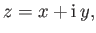(6.1)

where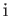represents the square root of minus one. Here,andare both real, and are identified with the corresponding Cartesian coordinates. (Incidentally,should not be confused with a-coordinate: this is a strictly two-dimensional discussion.) We can also write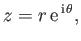(6.2)

where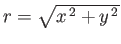and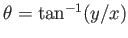are the modulus and argument of, respectively, but can also be identified with the corresponding plane polar coordinates. Finally, Euler's theorem (Riley 1974),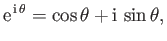(6.3)

implies that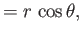(6.4)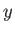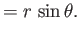(6.5)

We can define functions of the complex variable,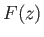, in the same way that we define functions of a real variable. For instance,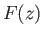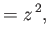(6.6)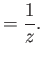(6.7)

For a given function,, we can substitute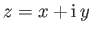and write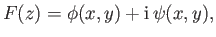(6.8)

where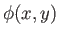andare real two-dimensional functions. Thus, if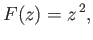(6.9)

then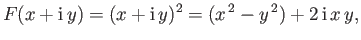(6.10)

giving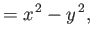(6.11)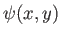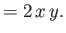(6.12)Next: Cauchy-Riemann Relations Up: Two-Dimensional Potential Flow Previous: Introduction
Richard Fitzpatrick 2016-03-31